### Circles Class 10th Mathematics CBSE Solution

##### Question 1.From a point Q, the length of the tangent to a circle is 24 cm and the distance of Q from the centre is 25 cm. The radius of the circle isA. 7 cm B. 12 cmC. 15 cm D. 24.5 cmAnswer:(A)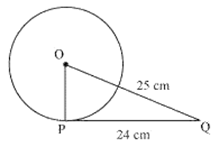Let O be the centre of the circle.Given that,OQ = 25cm and PQ = 24 cmAs the radius is perpendicular to the tangent at the point of contact,Therefore, OP ⊥ PQApplying Pythagoras theorem in ΔOPQ, we obtainOP2 + PQ2 = OQ2OP2 + 242 = 252OP2 = 625 - 576OP2 = 49OP =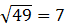Therefore, the radius of the circle is 7 cm.Question 2.In Fig. 10.11, if TP and TQ are the two tangents to a circle with centre O so that ∠ POQ = 110°, then ∠ PTQ is equal toA. 60° B. 70°C. 80° D. 90°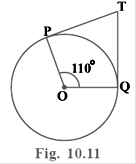Answer:Given: TP and TQ are tangents.Therefore, radius drawn to these tangents from centre of the circle will be perpendicular to the tangents.Thus, OP ⊥ TP and OQ ⊥ TQAnd therefore,∠OPT = 90°∠OQT = 90°In quadrilateral POQT,Sum of all interior angles = 360°∠OPT + ∠POQ +∠OQT + ∠PTQ = 360°90°+ 110° + 90° + ∠PTQ = 360°∠PTQ = 70°Question 3.If tangents PA and PB from a point P to a circle with centre O are inclined to each other at angle of 80°, then ∠ POA is equal toA. 50° B. 60°C. 70° D. 80°Answer:Given: PA and PB are tangents.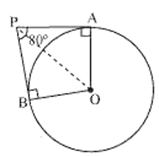Therefore, the radius drawn to these tangents will be perpendicular to the tangents.Thus, OA ⊥ PA and OB ⊥ PB∠OBP = 90°∠OAP = 90°In quadrilateral AOBP,Sum of all interior angles = 360°∠OAP + ∠APB +∠PBO + ∠BOA = 360° 90° + 80° +90° + ∠BOA = 360°∠BOA = 100°In ΔOPB and ΔOPA,AP = BP (Tangents from a point)OA = OB (Radii of the circle)OP = OP (Common side)Therefore, ΔOPB ≅ ΔOPA (SSS congruence criterion)And thus, ∠POB = ∠POA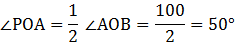Question 4.Prove that the tangents drawn at the ends of a diameter of a circle are parallel.Answer: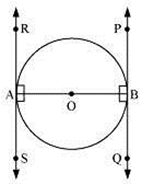Let AB is diameter of the circle. Two tangents PQ and RS are drawn at points A and B respectively. Radius drawn to these tangents will be perpendicular to the tangents.Thus, OA ⊥ RS and OB ⊥ PQ∠OAR = 90°∠OAS = 90°∠OBP = 90°∠OBQ = 90°It can be observed that∠OAR = ∠ OBQ (Alternate interior angles)∠OAS = ∠ OBP (Alternate interior angles)Since alternate interior angles are equal, lines PQ and RS will be parallel.Question 5.Prove that the perpendicular at the point of contact to the tangent to a circle passes through the centre.Answer:to Prove: A line drawn perpendicular from P will pass from O.Given: APB is tangent to circle with centre O.Let us consider a circle with centre O. Let AB be a tangent which touches the circle at P.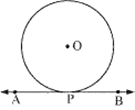Proof: Assume that the perpendicular to AB at P does not pass through centre O. Let it pass through another point O'. Join OP and O'P.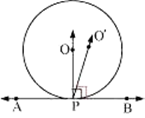As perpendicular to AB at P passes through O', therefore,∠ O'PB = 90° ... (1)O is the centre of the circle and P is the point of contact. We know the line joining the centre and the point of contact to the tangent of the circle are perpendicular to each other.∴ ∠ OPB = 90° ... (2)Comparing equations (1) and (2), we obtain∠ O'PB = ∠ OPB ... (3)From the figure, it can be observed that,∠ O'PB < ∠ OPB ... (4)Therefore, ∠ O'PB = ∠ OPB is not possible. It is only possible, when the line O'P coincides with OP.Therefore, the perpendicular to AB through P passes through centre O.Hence, Proved.Question 6.The length of a tangent from a point A at distance 5 cm from the centre of the circle is 4cm. Find the radius of the circle.Answer: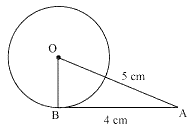Let us consider a circle centred at point O.AB is a tangent drawn on this circle from point A.Given that,OA = 5cm and AB = 4 cmIn ΔABO,OB ⊥ AB (Radius ⊥ tangent at the point of contact)Applying Pythagoras theorem in ΔABO, we obtainAB2 + BO2 = OA242 + BO2 = 5216 + BO2 = 25 BO2 = 9BO = 3Hence, the radius of the circle is 3 cm.Question 7.Two concentric circles are of radii 5 cm and 3 cm. Find the length of the chord of the larger circle which touches the smaller circle.Answer: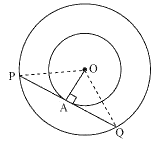Let the two concentric circles be centred at point O. And let PQ be the chord of the larger circle which touches the smaller circle at point A. Therefore, PQ is tangent to the smaller circle.OA ⊥ PQ (As OA is the radius of the circle)Applying Pythagoras theorem in ΔOAP, we obtainOA2 + AP2 = OP232 + AP2 = 529 + AP2 = 25AP2 = 16AP = 4In ΔOPQ,Since OA ⊥ PQ,AP = AQ (Perpendicular from the centre of the circle bisects the chord)PQ = 2AP = 2 × 4 = 8Therefore, the length of the chord of the larger circle is 8 cm.Question 8.A quadrilateral ABCD is drawn to circumscribe a circle (see Fig. 10.12). Prove thatAB + CD = AD + BC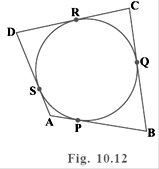Answer: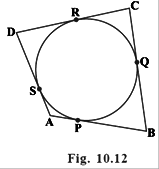To Prove: AB + CD = AD + BCProof:In the given figure, it can be observed that AB touches the circle at point P; BC touches the circle at point Q; CD touches the circle at point R; DA touches the circle at point S.Then, we can say from a theorem that " Length of tangents drawn from an external point to the circle are same ", Therefore,DR = DS (Tangents on the circle from point D) ….. (1)CR = CQ (Tangents on the circle from point C) …... (2)BP = BQ (Tangents on the circle from point B) ….... (3)AP = AS (Tangents on the circle from point A) ….... (4)Adding all these equations, we obtainDR + CR + BP + AP = DS + CQ + BQ + AS(DR + CR) + (BP + AP) = (DS + AS) + (CQ + BQ)CD + AB = AD + BC Hence, Proved.Question 9.In Fig. 10.13, XY and X′Y′ are two parallel tangents to a circle with centre O and another tangent AB with point of contact C intersecting XY at A and X′Y′ at B. Prove that ∠ AOB = 90°.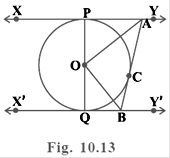Answer:Let us join point O to C.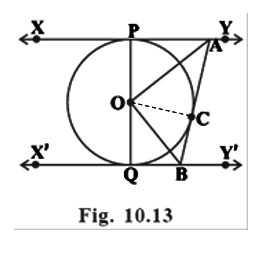In ΔOPA and ΔOCA,OP = OC (Radius of the same circle)AP = AC (Tangents from point A)AO = AO (Common side)ΔOPA ≅ ΔOCA (SSS congruence criterion)∠POA = ∠COA … (i)Similarly, ΔOQB ≅ ΔOCB∠QOB = ∠COB … (ii)Since POQ is a diameter of the circle, it is a straight line.Therefore, ∠POA + ∠COA + ∠COB + ∠QOB = 180°From equations (i) and (ii), it can be observed that 2∠COA + 2 ∠COB = 180°∠ COA + ∠ COB = 180° / 2∠COA + ∠COB = 90°∠AOB = 90°Hence Proved. Question 10.Prove that the angle between the two tangents drawn from an external point to a circle is supplementary to the angle subtended by the line segment joining the points of contact at the centre.Answer: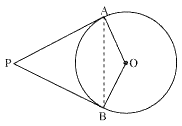To Prove: ∠ APB + ∠ BOA = 180°Proof: Let us consider a circle centred at point O.Let P be an external point from which two tangents PA and PB are drawn to the circle which touches the circle at point A and B respectively and AB is the line segment, joining point of contacts A and B together such that it subtends∠ AOB at centre O of the circle.It can be observed thatOA ⊥ PA (radius of circle is always perpendicular to tangent)Therefore, ∠ OAP = 90°Similarly, OB ⊥ PB∠ OBP = 90°In quadrilateral OAPB,Sum of all interior angles = 360°∠ OAP +∠ APB+∠ PBO +∠ BOA = 360°90° + ∠ APB + 90° + ∠ BOA = 360°∠ APB + ∠ BOA = 180°Hence, it can be observed that the angle between the two tangents drawn from an external point to a circle is supplementary to the angle subtended by the line-segment joining the points of contact at the centre.Question 11.Prove that the parallelogram circumscribing a circle is a rhombus.Answer:Since ABCD is a parallelogram,AB = CD ...(1)BC = AD ...(2)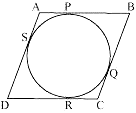It can be observed thatDR = DS (Tangents on the circle from point D)CR = CQ (Tangents on the circle from point C)BP = BQ (Tangents on the circle from point B)AP = AS (Tangents on the circle from point A)Adding all these equations, we obtainDR + CR + BP + AP = DS + CQ + BQ + AS(DR + CR) + (BP + AP) = (DS + AS) + (CQ + BQ)CD + AB = AD + BCOn putting the values of equations (1) and (2) in this equation, we obtain 2AB = 2BCAB = BC ...(3)Comparing equations (1), (2), and (3), we obtainAB = BC = CD = DAHence, ABCD is a rhombus.Question 12.A triangle ABC is drawn to circumscribe a circle of radius 4 cm such that the segments BD and DC into which BC is divided by the point of contact D are of lengths 8 cm and 6 cm respectively (see Fig. 10.14). Find the sides AB and AC.Answer: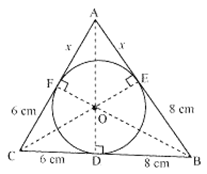To Find: AB and ACLet the given circle touch the sides AB and AC of the triangle at point E and F respectively and the length of the line segment AF be x.In ∆ABC,From the theorem which states that the lengths of two tangents drawn from an external point to a circle are equal.So, CF = CD = 6cm (Tangents on the circle from point C)BE = BD = 8cm (Tangents on the circle from point B)AE = AF = x (Tangents on the circle from point A)AB = AE + EB = x + 8BC = BD + DC = 8 + 6 = 14CA = CF + FA = 6 + xBy heron's formula,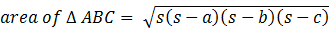where, a, b and c are sides of triangle and s is semi perimeter.2S = AB + BC + CA= x + 8 + 14 + 6 + x= 28 + 2xS =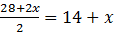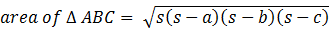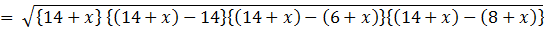=√(14 + x)(x)(8)(6)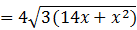Also, area of triangle =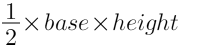And from the given figure it is clear thatArea of ΔABC = Area of ΔOBC + Area of ΔOCA + Area of ΔOABArea of ΔOBC =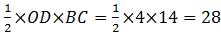Area of ΔOCA =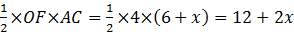Area of ΔOAB =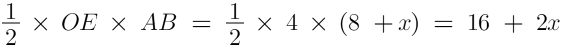Area of ΔABC = Area of ΔOBC + Area of ΔOCA + Area of ΔOAB⇒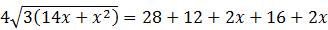⇒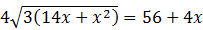⇒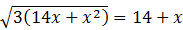⇒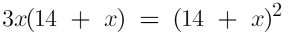⇒ 3x = 14 + x⇒ 2x = 14⇒ x = 7Therefore, x = 7Hence, AB = x + 8 = 7 + 8 = 15 cmCA = 6 + x = 6 + 7 = 13 cmHence, AB = 15 cm and AC = 13 cmQuestion 13.Prove that opposite sides of a quadrilateral circumscribing a circle subtend supplementary angles at the centre of the circle.Answer: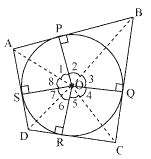To Prove : ∠ AOB + ∠ COD = 180°, ∠ BOC + ∠ DOA = 180°Given: ABCD is circumscribing the circle.Proof: Let ABCD be a quadrilateral circumscribing a circle centred at O such that it touches the circle at point P, Q, R, S.join the vertices of the quadrilateral ABCD to the centre of the circle.Consider ΔOAP and ΔOAS,AP = AS (Tangents from the same point)OP = OS (Radii of the same circle)OA = OA (Common side)ΔOAP ≅ ΔOAS (SSS congruence criterion)And thus, ∠ POA = ∠ AOS∠1 = ∠ 8 Similarly,∠2 = ∠ 3∠4 = ∠ 5∠6 = ∠ 7∠1 + ∠ 2 + ∠ 3 + ∠ 4 + ∠ 5 + ∠ 6 + ∠ 7 + ∠ 8 = 360°(∠ 1 + ∠ 8) + (∠ 2 + ∠ 3) + (∠ 4 + ∠ 5) + (∠ 6 + ∠ 7) = 360°2∠ 1 + 2∠ 2 + 2∠ 5 + 2∠ 6 = 360°2(∠ 1 + ∠ 2) + 2(∠ 5 + ∠ 6) = 360°(∠ 1 + ∠ 2) + (∠ 5 + ∠ 6) = 180°∠ AOB + ∠ COD = 180°Similarly, we can prove that ∠ BOC + ∠ DOA = 180°Hence, opposite sides of a quadrilateral circumscribing a circle subtend supplementary angles at the centre of the circle.

PDF FILE TO YOUR EMAIL IMMEDIATELY PURCHASE NOTES & PAPER SOLUTION. @ Rs. 50/- each (GST extra)

HINDI ENTIRE PAPER SOLUTION

MARATHI PAPER SOLUTION

SSC MATHS I PAPER SOLUTION

SSC MATHS II PAPER SOLUTION

SSC SCIENCE I PAPER SOLUTION

SSC SCIENCE II PAPER SOLUTION

SSC ENGLISH PAPER SOLUTION

SSC & HSC ENGLISH WRITING SKILL

HSC ACCOUNTS NOTES

HSC OCM NOTES

HSC ECONOMICS NOTES

HSC SECRETARIAL PRACTICE NOTES

# 2019 Board Paper Solution

HSC ENGLISH SET A 2019 21st February, 2019

HSC ENGLISH SET B 2019 21st February, 2019

HSC ENGLISH SET C 2019 21st February, 2019

HSC ENGLISH SET D 2019 21st February, 2019

SECRETARIAL PRACTICE (S.P) 2019 25th February, 2019

HSC XII PHYSICS 2019 25th February, 2019

CHEMISTRY XII HSC SOLUTION 27th, February, 2019

OCM PAPER SOLUTION 2019 27th, February, 2019

HSC MATHS PAPER SOLUTION COMMERCE, 2nd March, 2019

HSC MATHS PAPER SOLUTION SCIENCE 2nd, March, 2019

SSC ENGLISH STD 10 5TH MARCH, 2019.

HSC XII ACCOUNTS 2019 6th March, 2019

HSC XII BIOLOGY 2019 6TH March, 2019

HSC XII ECONOMICS 9Th March 2019

SSC Maths I March 2019 Solution 10th Standard11th, March, 2019

SSC MATHS II MARCH 2019 SOLUTION 10TH STD.13th March, 2019

SSC SCIENCE I MARCH 2019 SOLUTION 10TH STD. 15th March, 2019.

SSC SCIENCE II MARCH 2019 SOLUTION 10TH STD. 18th March, 2019.

SSC SOCIAL SCIENCE I MARCH 2019 SOLUTION20th March, 2019

SSC SOCIAL SCIENCE II MARCH 2019 SOLUTION, 22nd March, 2019

XII CBSE - BOARD - MARCH - 2019 ENGLISH - QP + SOLUTIONS, 2nd March, 2019

# HSCMaharashtraBoardPapers2020

(Std 12th English Medium)

HSC ECONOMICS MARCH 2020

HSC OCM MARCH 2020

HSC ACCOUNTS MARCH 2020

HSC S.P. MARCH 2020

HSC ENGLISH MARCH 2020

HSC HINDI MARCH 2020

HSC MARATHI MARCH 2020

HSC MATHS MARCH 2020

# SSCMaharashtraBoardPapers2020

(Std 10th English Medium)

English MARCH 2020

HindI MARCH 2020

Hindi (Composite) MARCH 2020

Marathi MARCH 2020

Mathematics (Paper 1) MARCH 2020

Mathematics (Paper 2) MARCH 2020

Sanskrit MARCH 2020

Sanskrit (Composite) MARCH 2020

Science (Paper 1) MARCH 2020

Science (Paper 2)

Geography Model Set 1 2020-2021

MUST REMEMBER THINGS on the day of Exam

Are you prepared? for English Grammar in Board Exam.

Paper Presentation In Board Exam

How to Score Good Marks in SSC Board Exams

Tips To Score More Than 90% Marks In 12th Board Exam

How to write English exams?

How to prepare for board exam when less time is left

How to memorise what you learn for board exam

No. 1 Simple Hack, you can try out, in preparing for Board Exam

How to Study for CBSE Class 10 Board Exams Subject Wise Tips?

JEE Main 2020 Registration Process – Exam Pattern & Important Dates

NEET UG 2020 Registration Process Exam Pattern & Important Dates

How can One Prepare for two Competitive Exams at the same time?

8 Proven Tips to Handle Anxiety before Exams!